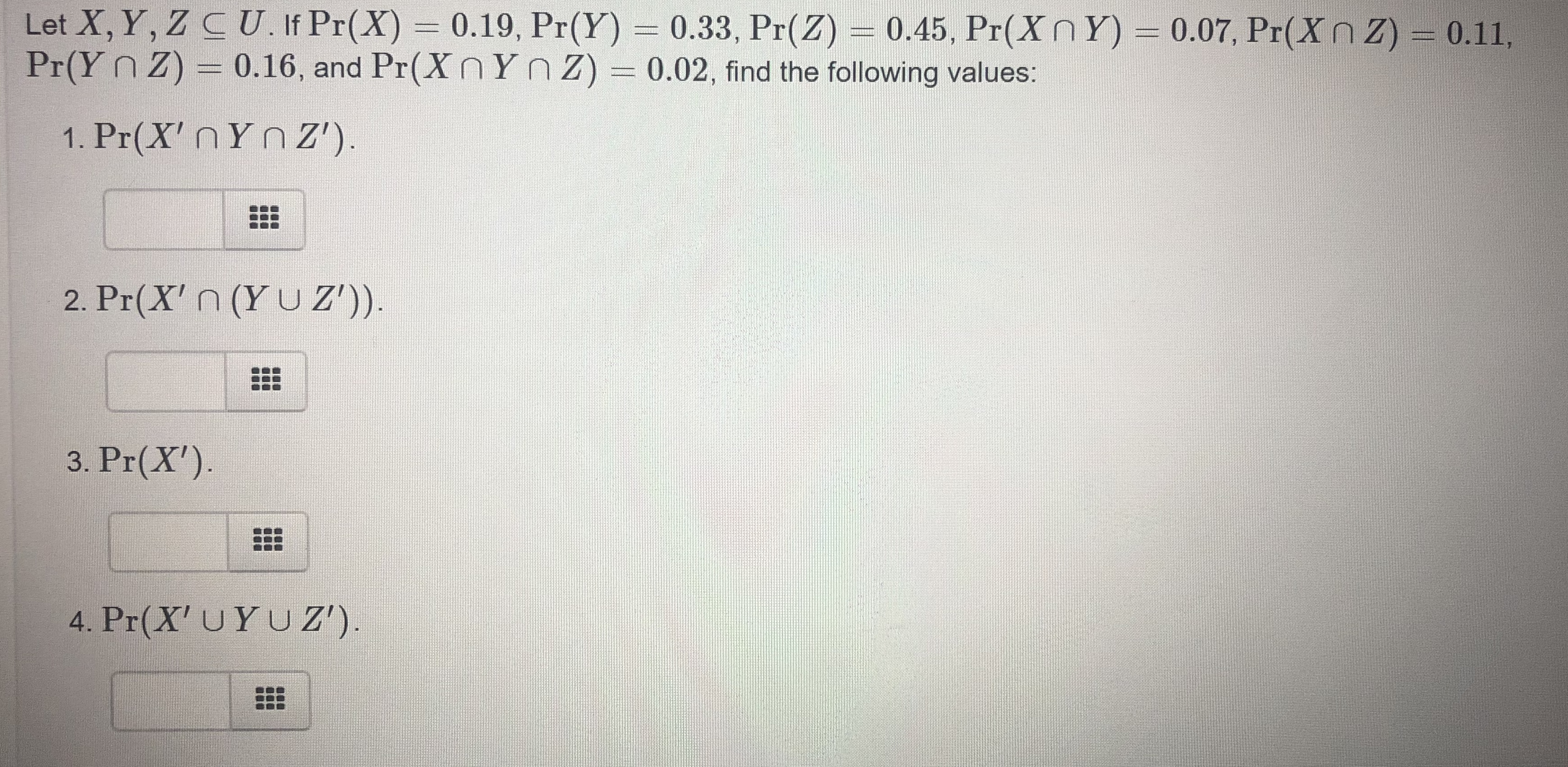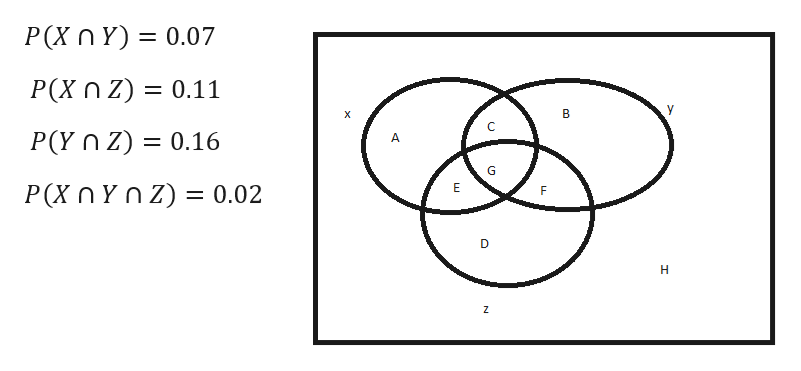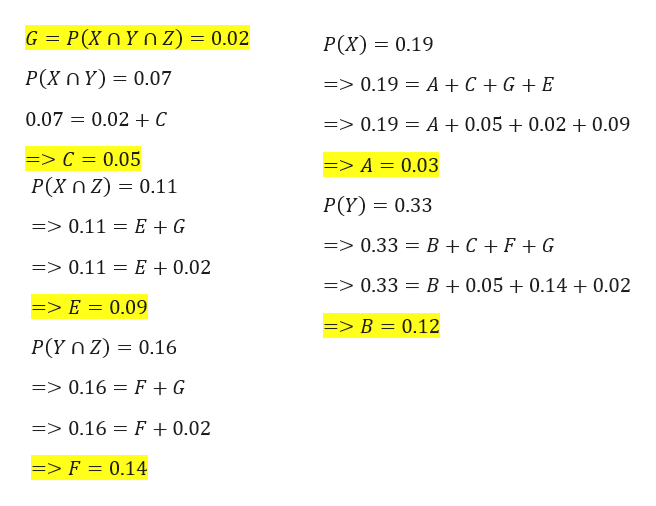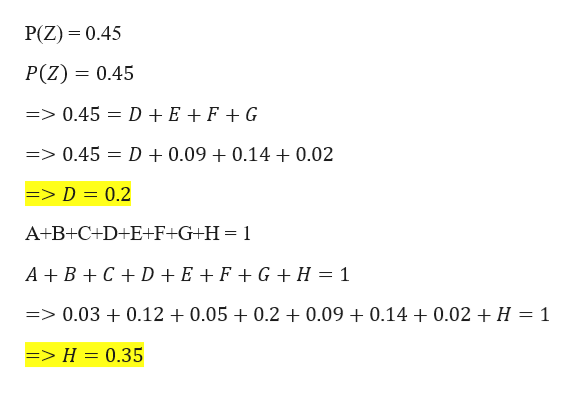# Let X, Y, Z C U. If Pr(X) 0.19, Pr(Y) 0.33, Pr(Z) 0.45, Pr(Xn Y) 0.07, Pr(Xn Z)Pr(Y n Z) 0.16, and Pr(X nYnz) = 0.02, find the following values:0.11,ddtit1. Pr(X' n Yn Z')2. Pr(X' n (Y U Z'))3. Pr(X')4. Pr(X' UYU Z')

Question
2 viewshelp_outlineImage TranscriptioncloseLet X, Y, Z C U. If Pr(X) 0.19, Pr(Y) 0.33, Pr(Z) 0.45, Pr(Xn Y) 0.07, Pr(Xn Z) Pr(Y n Z) 0.16, and Pr(X nYnz) = 0.02, find the following values: 0.11, ddtit 1. Pr(X' n Yn Z') 2. Pr(X' n (Y U Z')) 3. Pr(X') 4. Pr(X' UYU Z') fullscreen
check_circle

Step 1

Given Data
P(X) = 0.19
P(Y) = 0.33
P(Z) = 0.45help_outlineImage TranscriptioncloseP(X n Y) 0.07 11 0 P(X n z) 0.11 B X P(Y n Z) 0.16 A G P(X n Yn z) 0.02 E D н Z ш fullscreen
Step 2

Calculations From The Venn diagram shown below
A+B+C+D+E+F+G+H = 1help_outlineImage TranscriptioncloseG = P(XnYn Z) = 0.02 P(X) 0.19 P(X n Y) 0.07 => 0.19 A +C +GE 0.07 0.02 +C A +0.05 0.02 0.09 => 0.19 => C = 0.05 => A = 0.03 P(Xn z) 0.11 P(Y) 0.33 => 0.11 E + G => 0.33 B + C F + G => 0.11 E +0.02 => 0.33 B + 0.05 + 0.14 +0.02 => E = 0.09 => B = 0.12 P(Y n z) 0.16 => 0.16 F +G => 0.16 F +0.02 => F = 0.14 fullscreen
Step 3

Calculation Co...help_outlineImage TranscriptioncloseP(Z) 0.45 P(Z) 0.45 D +E + F + G => 0.45 D +0.09 + 0.14 +0.02 => 0.45 => D = 0.2 A+B+C+D+E+F+G+H=1 A+BCD +E +F +G H 1 0.09 + 0.14 + 0.02 + H = 1 => 0.03 0.12 0.05 + 0.2 => H = 0.35 fullscreen

### Want to see the full answer?

See Solution

#### Want to see this answer and more?

Solutions are written by subject experts who are available 24/7. Questions are typically answered within 1 hour.*

See Solution
*Response times may vary by subject and question.
Tagged in

### Other